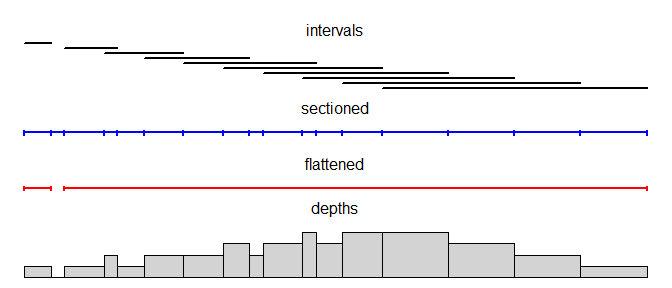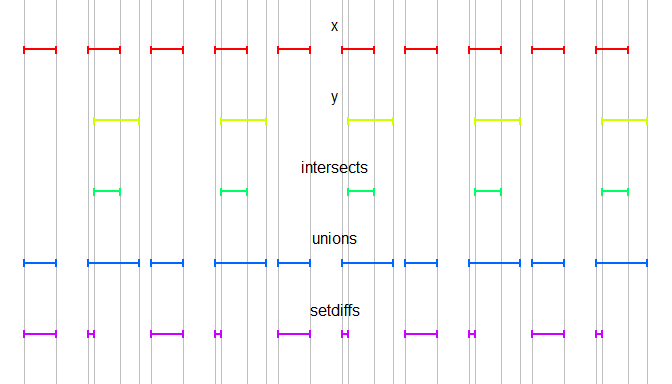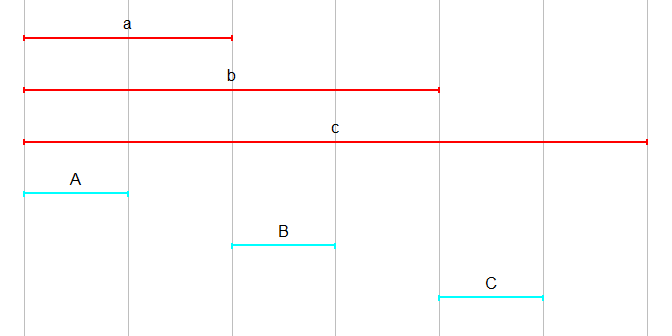IntervalSurgeon

Introduction

IntervalSurgeon presents functions for intersecting, overlapping, piling and annotating integer-bounded intervals. Underlying algorithms are written in C++ for efficiency where appropriate (with the help of the Rcpp package). A typical use case would be for manipulating genomic intervals.

For the purposes of this package, intervals are represented as two-column integer matrices where the inclusive start points are in the first column and the exclusive end points are in the second column.

library(IntervalSurgeon)

starts <- 3*1:10
ends <- 5*1:10

intervals <- cbind(starts, ends)

The lengths of the intervals are therefore: intervals[,2]-intervals[,1].

A key concept in IntervalSurgeon is breaking ranges which contain intervals into 'sections' delimited by the unique start/end points in the set. The sections for a set of intervals x is therefore a two-column matrix representing a set of intervals which partitions the range of x by the sorted start and end points. The sorted start and end points can be obtained using the breaks function (so named to reflect start/end points of intervals frequently being referred to as 'breakpoints' in genomics), which is equivalent to: sort(unique(as.integer(intervals)))). The sections can be computed from the sorted start and end points using the sections function.

One can use the depth and pile functions respectively to obtain the depth of intervals over their 'sections' (within which the depth is constant), and the row indices of original intervals which cover each section.

The flatten function returns a non-touching, non-overlapping set of intervals (as a matrix) which overlap at least one of a given set.

sectioned <- sections(breaks(intervals))
flattened <- flatten(intervals)
depths <- depth(intervals)
piles <- pile(intervals)Detached intervals

IntervalSurgeon provides functions which are optimised for dealing with detached (i.e. non-overlapping and non-touching) intervals which are sorted and non-empty. Here, we generate two such sets of intervals:

x_starts <- 10*1:10
x <- cbind(x_starts, x_starts + 5)

y_starts <- 20*1:5 + 1
y <- cbind(y_starts, y_starts + 7)

We can determine that they are indeed detached, sorted and non-empty using the detached_sorted_nonempty function.

detached_sorted_nonempty(x)
##  TRUE

The IntervalSurgeon functions for operating on such sets of detached, sorted, non-empty intervals are analogues of the set operation functions in the base package, namely: intersect, union and setdiff. Here, the function names are in plural (i.e. with extra s's on the end).For a given set of sorted, non-overlapping intervals, some of the start points might be the same as the end points of adjacent intervals. These intervals are therefore 'touching' and can be 'stitched' together using the stitch function. If there were overlaps, the flatten function can be used to generate a set of sorted disjoint intervals. Note that flatten will also stitch touching intervals, although the stitch function is faster (albeit requiring intervals to be sorted).

Finding overlaps between sets of intervals

Information about overlaps between sets of intervals can be obtained by 'joining' the sets. This is analogous to an SQL inner-join of two tables, and can be performed on sets of intervals using the join function. This function returns a matrix containing all overlaps of intervals from each set. Each row in the returned matrix corresponds to a specific overlap of intervals with one from each of the sets passed to the function. The nth element in a row contains the row index of the interval in the nth set of intervals passed to the function. Depending on the value of the output argument, there may two additional columns giving the start and end coordinates of the overlap (the default: output="intervals", no extra columns (output="indices") or one additional column giving the row index of the 'section' of the complete set of intervals (output="sections", see ?sections).

x <- cbind(3*1:8, 5*1:8)
y <- cbind(4*1:4, 7*1:4)
join_xy <- join(x, y)

##      [,1] [,2] [,3] [,4]
## [1,]    1    1    4    5
## [2,]    2    1    6    7
## [3,]    2    2    8   10
## [4,]    3    2    9   14
## [5,]    3    3   12   15
## [6,]    4    2   12   14

One common task would be to tag intervals with overlapping intervals, perhaps from a different set. For example, this might be useful for tagging a set of genomic intervals with the names of genes which they overlap. The annotate function is provided for this exact purpose.

x <- cbind(0, c(a=10, b=20, c=30))
y <- cbind(c(A=0, B=10, C=20), c(5, 15, 25))annotate(x, y)
## $a ##  "A" ## ##$b
##  "A" "B"
##
## $c ##  "A" "B" "C" Use with genomic intervals Genomic intervals are often represented in R as data.frames with columns corresponding to chromosome name, start position and end position. Obviously intervals do not intersect if they are on different chromosomes, so in order to manipulate such intervals with IntervalSurgeon, genome-wide operations must be performed chromosome-at-a-time. Using split to create a list of chromosome specific data.frames, or looping over the names of chromosomes and subsetting the original table, before cbinding/as.matrixing the start and end columns then makes the data accessible to the IntervalSurgeon functions. regions_annotated_with_genes <- lapply( c(1:22, "X", "Y"), function(chromosome) { regions_on_chr <- as.matrix(regions[regions$chr == chromosome,c("start", "end")])
genes_on_chr <- as.matrix(genes[genes\$chr == chromosome,c("start","end")])
annotate(regions_on_chr, genes_on_chr)
}
)